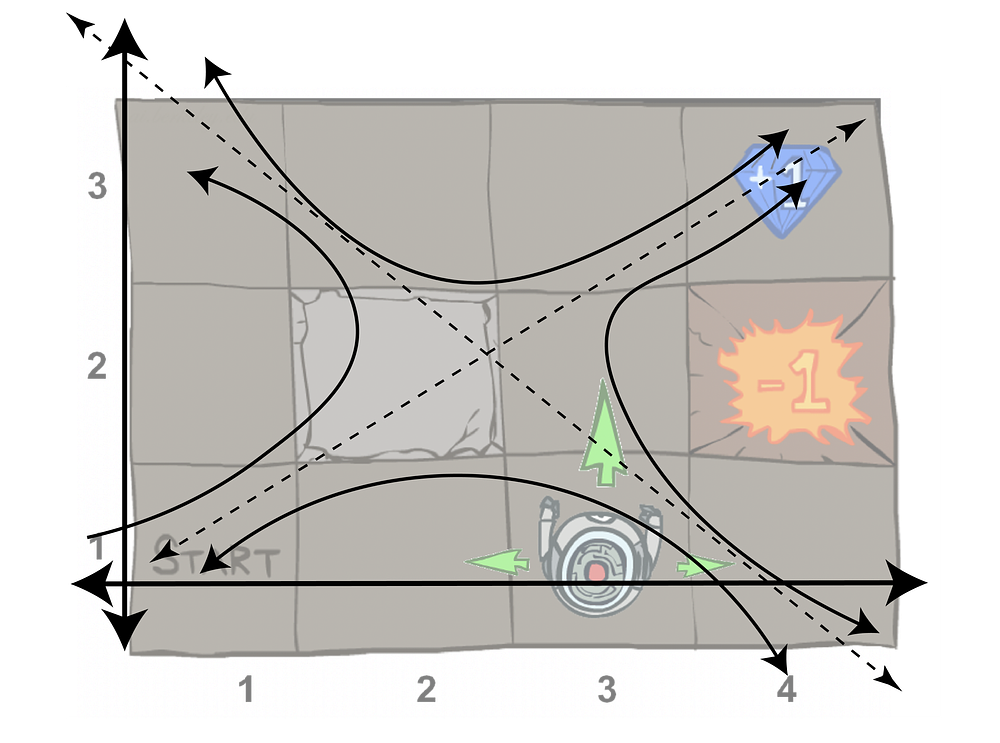# The hidden linear algebra of reinforcement learning

Original article can be found here (source): Artificial Intelligence on MediumRichard E. Bellman was a mathematician that laid the groundwork for modern control and optimization theory. Through a recursive one-step equation, a Bellman Update Equation, large optimization problems can be solved efficiently. With a recursive Bellman update, one can set up an optimization or control problem with Dynamic Programming, which is a process of creating smaller, more computationally tractable problems. This process proceeds recursively from the end — a receding horizon approach.

1. Bellman Equation: Necessary condition for optimality in optimization problems formulated as Dynamic Programming.
2. Dynamic Programing: Process to simplify an optimization problem by breaking it down into an optimal substructure.

In reinforcement learning, we use the Bellman Update process to solve for the optimal values and q-values of a state-action space. This is ultimately formulating the expected sum of future rewards from a given location.

Here, we can see all of the values from the review interleaving. The notation (*) denotes optimal, so true or converged. We have the value of the state being determined by the best action, and a q-state, then two recursive definitions. The recursive values balance the probability of visiting any state in T(s,a,s’) and the reward of any transition R(s,a,s’) to create a global map for values of the state-action space.

They key point here is that we are multiplying matrices (R, T), by vectors (V,U), to iteratively solve for convergence. The values will converge from any initial state because of how the values for one state are determined by their neighbors s’. For more on MDPs — see this introduction I wrote.

## Reinforcement Learning?

“I was told there would be RL,” — you, reader, 4 minutes in. This is all reinforcement learning, and I assert understanding the assumptions and the model that the algorithms are built on will prepare you vastly better then just copying python tutorials from OpenAI. Do that after. I’ve mentored multiple students into working in RL, and the ones who get more done are always the ones that learn what is going on, and then how to apply it.

That being said, this is one small step away from online q-learning, where we estimate the same Bellman updates with samples of T and R rather than explicitly using them in the equations. All the same assertions apply, but it is over probability distributions and expectations. Q-learning is the famous algorithm that solved Atari games and more in ~2015.

# Hidden Math

## Eigenvalues? Huh.

Recall an eigenvalue-eigenvector pair (λ, u) of a system A is a vector and scalar such that the vector acted on by the system returns a scalar multiple of the original vector.

The beautiful thing about eigenvalues and eigenvectors is that when they span the state space (which they are guaranteed to do for most physical systems by something called generalized eigenvectors), every vector can be written as a combination of the other eigenvectors. Then, in discrete systems Eigenvectors control the evolutions from any initial state — any initial vector will combine to a linear combination of the eigenvectors.

## Stochastic Matrices and Markov Chains

MDPs are very close to, but not the same in structure to Markov Chains. Markov chains are determined by transition matrix P. The probability matrix acts like the transition matrix T(s,a,s’) summed over the actions. In Markov Chains, the next state is determined by:

This matrix P has some special values — you can see that this is an eigenvalue equation with all the eigenvalues equal to one (picture a λ=1 pre-multiplying the left side of the equation). In order to get a matrix guaranteed to have eigenvalues equal to one, all the columns must sum up to 1.

What we are looking for in RL now, is how does the evolution of our solutions relate to convergence of probability distributions? We do this by formulating the iterative operators for V* and Q* as a linear operator (a Matrix) B. Convergence can be tricky — the value and q-value vectors we use are not the eigenvectors — they converge to the eigenvectors, but that’s not important to seeing how eigenvectors govern the system.